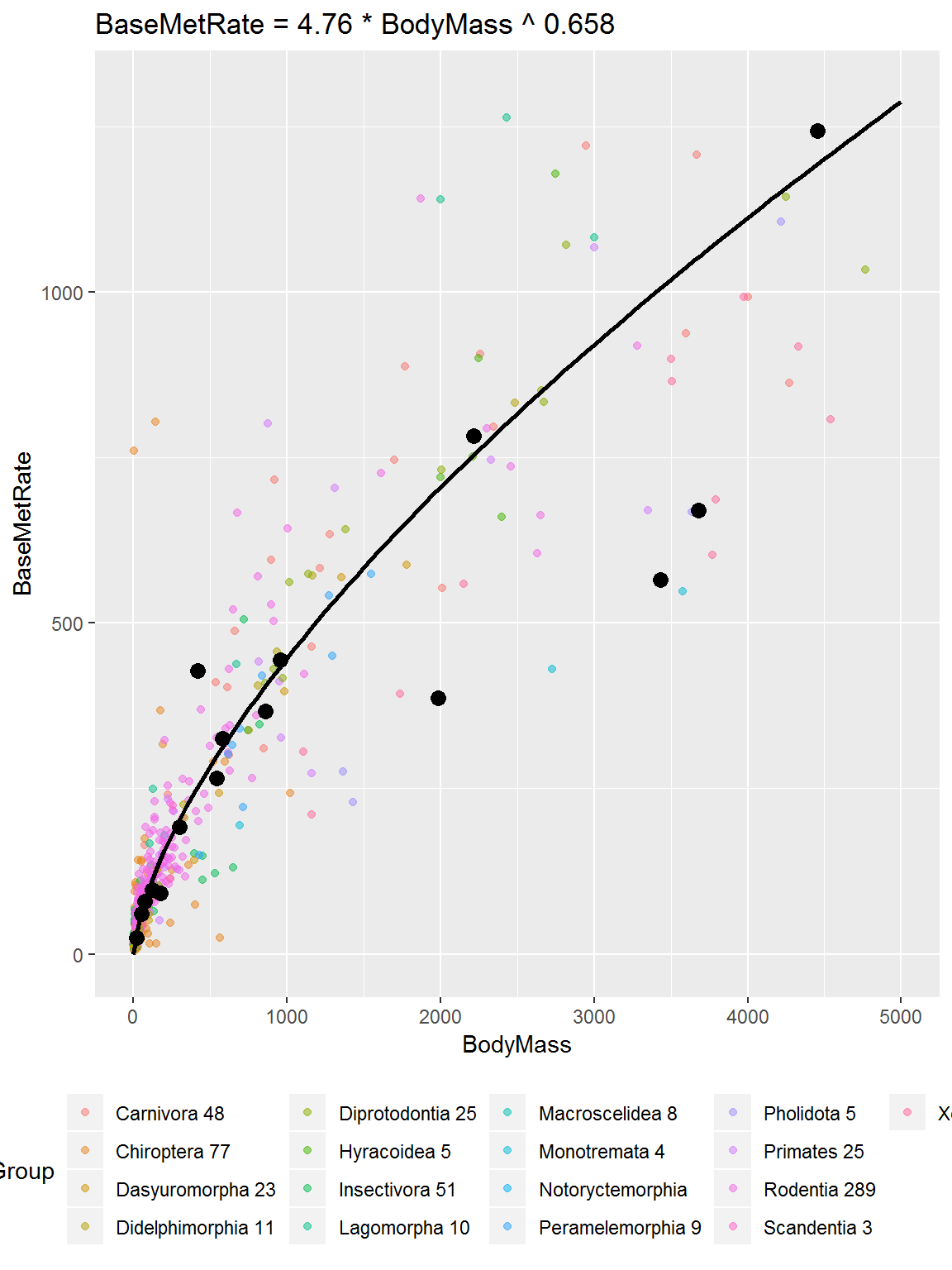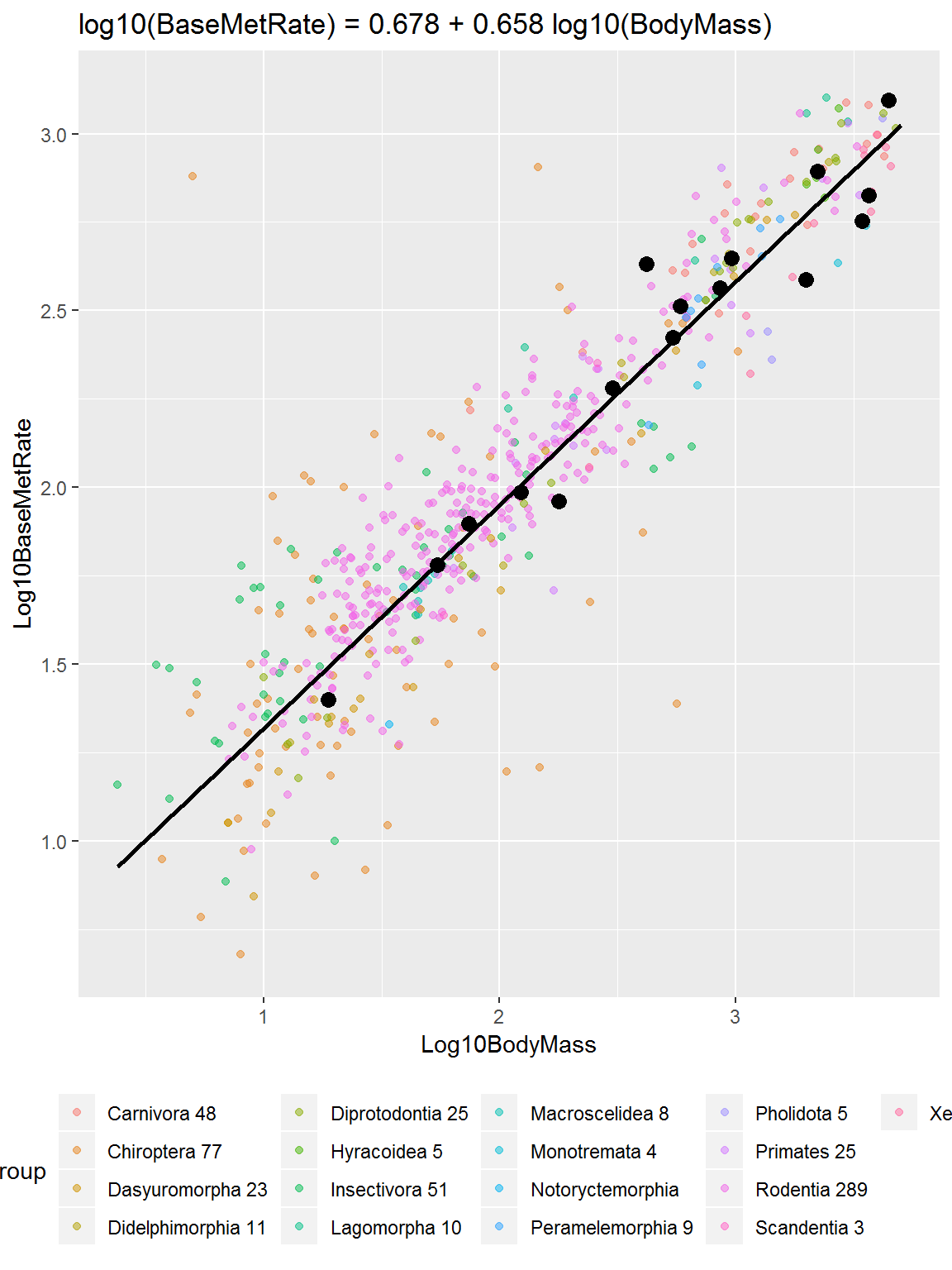# Example: Log-log transformation: metabolic rates

A rich source of examples when covering log-log transformations are biological scaling relations (one of the world experts in allometric scaling is Prof. Jim Brown, UNM Biology, http://biology.unm.edu/jhbrown). The relationship between body mass (M, g) and basal metabolic rate (BMR, ml of O\(_2\) per h (similar to Watts?)) for mammalian orders for selected data are summarized in plots below, both on the original and log-log scales (White and Seymour (2003) PNAS, 10.1073/pnas.0436428100). The linear regression summarizes the dark points, the mean for each of the species groups, and the colored points are individual species. The curved regression is found by inverting the linear regression onto the original scale. The third plot displays the log axes with values on the original scale.

``library(tidyverse)``
``## -- Attaching packages ------------- tidyverse 1.2.1 --``
``````## v ggplot2 3.1.0       v purrr   0.3.2
## v tibble  2.1.1       v dplyr   0.8.0.1
## v tidyr   0.8.3       v stringr 1.4.0
## v readr   1.3.1       v forcats 0.4.0``````
``````## -- Conflicts ---------------- tidyverse_conflicts() --
``````# http://www.ncbi.nlm.nih.gov/pmc/articles/PMC153045/
# Supp:
# http://www.ncbi.nlm.nih.gov/pmc/articles/PMC153045/bin/pnas_0436428100_index.html
# Supporting information for White and Seymour (2003)
#   Proc. Natl. Acad. Sci. USA, 10.1073/pnas.0436428100

library(gdata)``````
``## gdata: read.xls support for 'XLS' (Excel 97-2004) files ENABLED.``
``## ``
``## gdata: read.xls support for 'XLSX' (Excel 2007+) files ENABLED.``
``````##
## Attaching package: 'gdata'``````
``````## The following objects are masked from 'package:dplyr':
##
##     combine, first, last``````
``````## The following object is masked from 'package:purrr':
##
##     keep``````
``````## The following object is masked from 'package:stats':
##
##     nobs``````
``````## The following object is masked from 'package:utils':
##
##     object.size``````
``````## The following object is masked from 'package:base':
##
##     startsWith``````
``````fn <- "S4R_WS_23b_data_log-logScaling_BodyMassMetabolicRate_2003_WhiteSeymour.xlsx"
bm.bmr <- read.xls(fn, skip = 4)
bm.bmr\$Log10BodyMass <- log10(bm.bmr\$BodyMass)
bm.bmr\$Log10BaseMetRate <- log10(bm.bmr\$BaseMetRate)

# remove a very strange group
bm.bmr <- subset(bm.bmr, !(Group == "Artiodactyla 7"))
str(bm.bmr)``````
``````## 'data.frame':    634 obs. of  9 variables:
##  \$ Group           : Factor w/ 18 levels "Artiodactyla 7",..: 2 2 2 2 2 2 2 2 2 2 ...
##  \$ Genus           : Factor w/ 88 levels "","Acrobatidae",..: 1 12 12 12 12 12 12 12 12 31 ...
##  \$ Species         : Factor w/ 621 levels "","2n = 52","2n = 54",..: 1 22 67 68 79 206 614 615 616 8 ...
##  \$ BodyMass        : num  4452 3600 10000 7720 5444 ...
##  \$ T               : num  37.5 38.6 37 38 38.2 38.8 38 38.7 NA 39 ...
##  \$ BaseMetRate     : num  1244 1374 2687 3860 1524 ...
##  \$ Ref             : Factor w/ 239 levels "","1","10","100",..: 1 230 3 15 24 37 3 50 61 72 ...
##  \$ Log10BodyMass   : num  3.65 3.56 4 3.89 3.74 ...
##  \$ Log10BaseMetRate: num  3.09 3.14 3.43 3.59 3.18 ...``````
``````# log-log scale linear regression
lm.fit <- lm(Log10BaseMetRate ~ Log10BodyMass, data = bm.bmr)
# coefficients for regression line
coef(lm.fit)``````
``````##   (Intercept) Log10BodyMass
##     0.6775600     0.6575572``````
``````library(ggplot2)

p1 <- ggplot(subset(bm.bmr, (Genus == "")), aes(x = BodyMass, y = BaseMetRate))
p1 <- p1 + geom_point(data = subset(bm.bmr, !(Genus == "")), aes(colour = Group), alpha = 0.5)
p1 <- p1 + geom_point(size = 3)
# Using a custom function
f.org.scale <- function(BodyMass) { 10^coef(lm.fit) * BodyMass ^ coef(lm.fit)}
p1 <- p1 + stat_function(fun = f.org.scale, size = 1)
p1 <- p1 + labs(title = paste("BaseMetRate = ", signif(10^coef(lm.fit), 3), " * ", "BodyMass ^ ", signif(coef(lm.fit), 3), sep = ""))
p1 <- p1 + scale_y_continuous(limits=c(0, 1300))
p1 <- p1 + scale_x_continuous(limits=c(0, 5000))
p1 <- p1 + theme(legend.position = "bottom")
print(p1)``````
``## Warning: Removed 56 rows containing missing values (geom_point).``
``## Warning: Removed 5 rows containing missing values (geom_point).````````p2 <- ggplot(subset(bm.bmr, (Genus == "")), aes(x = Log10BodyMass, y = Log10BaseMetRate))
p2 <- p2 + geom_point(data = subset(bm.bmr, !(Genus == "")), aes(colour = Group), alpha = 0.5)
p2 <- p2 + geom_point(size = 3)
p2 <- p2 + geom_smooth(method = lm, se = FALSE, fullrange = TRUE, size = 1, colour = "black")
p2 <- p2 + labs(title = paste("log10(BaseMetRate) = ", signif(coef(lm.fit), 3), " + ", signif(coef(lm.fit), 3), " log10(BodyMass)", sep = ""))
p2 <- p2 + scale_y_continuous(limits=c(NA, log10(1300)))
p2 <- p2 + scale_x_continuous(limits=c(NA, log10(5000)))
p2 <- p2 + theme(legend.position = "bottom")
print(p2)``````
``## Warning: Removed 5 rows containing non-finite values (stat_smooth).``
``## Warning: Removed 56 rows containing missing values (geom_point).``
``## Warning: Removed 5 rows containing missing values (geom_point).````````p3 <- ggplot(subset(bm.bmr, (Genus == "")), aes(x = BodyMass, y = BaseMetRate))
p3 <- p3 + geom_point(data = subset(bm.bmr, !(Genus == "")), aes(colour = Group), alpha = 0.5)
p3 <- p3 + geom_point(size = 3)
p3 <- p3 + geom_smooth(method = lm, se = FALSE, fullrange = TRUE, size = 1, colour = "black")
p3 <- p3 + labs(title = paste("log10(BaseMetRate) = ", signif(coef(lm.fit), 3), " + ", signif(coef(lm.fit), 3), " log10(BodyMass)", sep = ""))
p3 <- p3 + scale_y_log10()#limits=c(NA, log10(1300)))
p3 <- p3 + scale_x_log10()#limits=c(NA, log10(5000)))
p3 <- p3 + theme(legend.position = "bottom")
print(p3)``````
``## Warning: Removed 5 rows containing non-finite values (stat_smooth).``
``## Warning: Removed 1 rows containing missing values (geom_point).``
``## Warning: Removed 5 rows containing missing values (geom_point).``